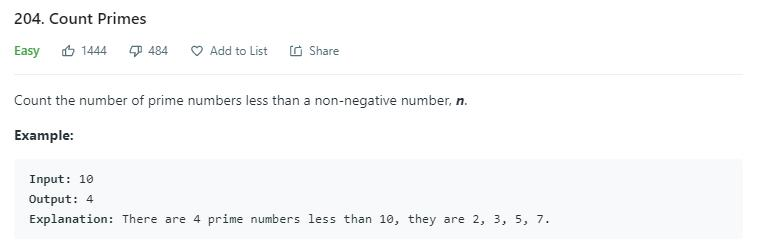# 题目描述（简单难度）# 解法一

public int countPrimes(int n) {
int count = 0;
for (int i = 2; i < n; i++) {
if (isPrime(i)) {
count++;
}
}
return count;
}

private boolean isPrime(int n) {
int sqrt = (int) Math.sqrt(n);
for (int i = 2; i <= sqrt; i++) {
if (n % i == 0) {
return false;
}
}
return true;
}


# 解法二

public int countPrimes(int n) {
boolean[] notPrime = new boolean[n];
int count = 0;
for (int i = 2; i < n; i++) {
if (!notPrime[i]) {
count++;
//将当前素数的倍数依次标记为非素数
for (int j = 2; j * i < n; j++) {
notPrime[j * i] = true;
}
}
}
return count;
}